×
Get Full Access to University Physics - 13 Edition - Chapter 2 - Problem 9e
Get Full Access to University Physics - 13 Edition - Chapter 2 - Problem 9e

×

# A ball moves in a straight line (the x-axis). The graph inISBN: 9780321675460 31

## Solution for problem 9E Chapter 2

University Physics | 13th Edition

• Textbook Solutions
• 2901 Step-by-step solutions solved by professors and subject experts
• Get 24/7 help from StudySoup virtual teaching assistantsUniversity Physics | 13th Edition

4 5 1 261 Reviews
21
1
Problem 9E

A ball moves in a straight line (the x-axis). The graph in Fig. E2.9 shows this ball’s velocity as a function of time. (a) What are the ball’s average speed and average velocity during the first 3.0 s? (b) Suppose that the ball moved in such a way that the graph segment after 2.0 s was -3.0 m/s instead of +3.0 m/s. Find the ball’s average speed and average velocity in this case.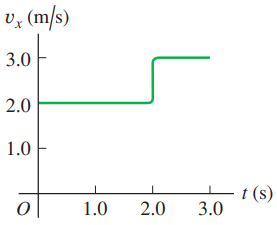Step-by-Step Solution:

Step 1 of 4

a)

Average speed, s = Total distance travelled by the object/Total time.

In this problem, the velocity of the ball in first two seconds is,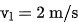.

Therefore, the total distance travelled by the object at first two seconds,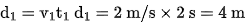The total distance travelled by the object in between 2nd and 3rd second is,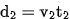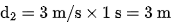Therefore, the total distance travelled by the object,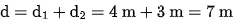Total time taken,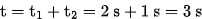Average speed of the ball, s = 7 m / 3 s = 2.33 m/s

Step 2 of 3

Step 3 of 3

##### ISBN: 9780321675460

The full step-by-step solution to problem: 9E from chapter: 2 was answered by , our top Physics solution expert on 05/06/17, 06:07PM. Since the solution to 9E from 2 chapter was answered, more than 6585 students have viewed the full step-by-step answer. This full solution covers the following key subjects: ball, average, velocity, speed, graph. This expansive textbook survival guide covers 26 chapters, and 2929 solutions. The answer to “?A ball moves in a straight line (the x-axis). The graph in Fig. E2.9 shows this ball’s velocity as a function of time. (a) What are the ball’s average speed and average velocity during the first 3.0 s? (b) Suppose that the ball moved in such a way that the graph segment after 2.0 s was -3.0 m/s instead of +3.0 m/s. Find the ball’s average speed and average velocity in this case.” is broken down into a number of easy to follow steps, and 73 words. University Physics was written by and is associated to the ISBN: 9780321675460. This textbook survival guide was created for the textbook: University Physics, edition: 13.

Unlock Textbook Solution